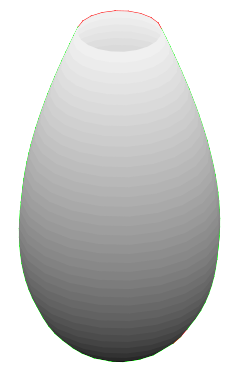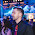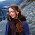Friday, May 16, 2014

The perfect shape

A liquid container designed to keep its content cool as long as possible would be spherical in shape, since the sphere is the closed surface that has the minimum area to enclosed volume ratio, thus minimizing convection heating. When the container is also meant to drink from, the sphere is no longer the optimum shape for coolness (leaving aside the fact that one should have to pierce it to access the liquid) because the surface of the remaining liquid changes as the container is being emptied.
A volume V of liquid at temperature T inside a glass is heated by convection on two different surfaces, one in direct contact with the environment and the other as enclosed by the glass wall.
These two surfaces contribute to the increase in temperature, given by Newton's law of cooling (here heating):
dT = (1/cvV)(hopen Aopen + hclosed Aclosed)(Tenv - T) dt,
where cv is the volumetric heat capacity of the liquid, hopen and hclosed the overall heat transfer coefficients of the open and closed interfaces, respectively, and Tenv the temperature of the environment. If the liquid is drunk at a constant rate of ϕ units of volume per unit of time, we have
dT = (1/cvϕV)(hopen Aopen + hclosed Aclosed)(T - Tenv) dV,
with Aopen and Aclosed varying as functions of V; this differential equation implicitly gives T as a function of (Aopen, Aclosed, V), although its analytical solution is only feasible for the simplest shapes. We define the optimum container as that for which the average temperature of the liquid during consumption
Tavg = [Vinit,0]TdV
is minimum.
Let us calculate this shape numerically. Candidate glasses are restricted to surfaces of revolution generated by nonnegative functions y = f(x) between 0 and some maximum height h.
We fix the rest of parameters: the contained liquid is one liter of water (or beer, for that matter) brought out of the fridge at 5 ºC and drunk in 3 minutes in a very hot summer afternoon at 40 ºC; the glass wall (made of, well, glass) is set to be 3 mm thick. All of this gives us:
Vinit = 1,000 cm3,
ϕ = 1,000/180 cm3/s,
Tinit = 5 ºC,
Tenv = 40 ºC,
cv = 4.1796 J/cm3/K,
hwater = 50 W/m2/K,
hair = 100 W/m2/K,
θglass = 3 mm,
kglass0.85 W/m/K,
hopen = 1/((1/hwater)+(1/hair)) = 33.3 W/m2/K,
hclosed = 1/((1/hwater)+(θglass/kglass)+(1/hair)) = 29.8 W/m2/K.
We will use a genetic algorithm to solve the problem:
• Candidate solutions are codified as arrays with the values f(0), f(0.5 cm), ... , f(50 cm) (that is, the maximum height of the glass is h = 50 cm, much higher than really needed —excess height will simply manifest as a tiny column of radius ≈ 0).
• The initial pool of candidates is populated with values from random Hermite interpolation polynomials of degree 3 normalized so that the enclosed volume is Vinit.
• Individual fitness is simply its Tavg calculated figure: the lower Tavg the fitter the solution.
• At each generation, the fittest 25% of the pool is kept and the rest replaced by random breeding of the former. Crossover of f0 and f1 is implemented by randomly selecting two cutoff points c1 and c2, putting f as:
• f(x) = f0(x) for x < c1,
• f(x) = f0(x)·(c2- x)/(c2- c1) + f1(x)·(x - c1)/(c2- c1) for c1x < c2,
• f(x) = f1(x) for xc2,
and normalizing f.
• Bred individuals are mutated (before normalization) by multiplying some of their values (mutation rate = 1%) by a random factor in the range [0,2).
The calculation program has been implemented in C++ (Boost, and in particular Boost.Units, is used). Trials with different initial populations and algorithm parameters (mutation rates, pool sizes and so on) yield basically the same result, which is depicted in the figure:Optimum glass profile.
The area of this glass is 537 cm2 and its associated average temp Tavg is 6.40 ºC (quite cool!). As predicted, the excess height from ~25 cm up shows as a tiny filament whose actual capacity is totally negligible: trimming the glass cap off at a height of 18.5 cm produces a more practical glass with an opening diameter of 4.2 cm and almost the same performance (we only dropped 2% of the initial content and the temperature of the liquid at that point, 3.6 seconds after beginning to drink, is only slightly higher than Tinit). The glass thus obtained is, perhaps surprisingly, very reminiscent of real-life glasses:Optimum glass trimmed at 18.5 cm.
In general, trimming the optimum glass f(x) for a given Tinit at height hcut produces the optimum solution of the problem for Vinit = V(height = hcut), Tinit = T(height = hcut) with the additional constraint that the opening of the glass is precisely 2f(hcut): we can then play with this property to obtain solutions to the modified problem
minimize Tavg while maintaining a given opening diameter δ
by starting with Vinit+ ΔV and Tinit -  ΔT and adjusting ΔV and ΔT until the volume and temperature at the desired opening hit Vinit and Tinit simultaneously (experimenting with this is left as an exercise for the reader).
Parameter sensitivity
An informal analysis reveals that the optimum glass shape is quite independent of changes in most problem parameters. In particular:
• Tinit does not affect significantly the solution (as long as Tinit < Tenv).
• Changing Vinit simply produces a scaled version of the same shape.
• Decreasing ϕ (that is, taking longer to drink the beer up) yields only slightly different shapes, with a wider lower segment.
The one aspect that truly changes the shape is the hopen/hclosed ratio. As this increases, the optimum glass gets thinner at the base and longer, approaching a cylinder as hopen/hclosed → ∞. This has practical implications: for instance, making the beer grow a thick foam topping reduces hopen, which allows for shorter, wider, rounder glasses.
Postscript
Some readers have asked why we haven't used calculus of variations to solve the problem. In its classical one-dimensional formulation (Euler-Lagrange equation), this calculus provides tools for finding the stationary values of a functional J(f) defined as
J(f) = [a,b] L(f(x), f'(x), x) dx,
for some function L : ℝ3 → ℝ with appropriate smoothness properties. The important aspect to note here is that L must depend on the point values of f and f' at x alone: by contrast, in our case the integrand T(V) is a function of hopen Aopen(v) + hclosed Aclosed(v) for all values of v in [V, Vinit], so the conditions for the application of Euler-Lagrange equation do not hold.

1.Would be interesting to know whether this was a feature ancient people's approximated when transporting amphora of various shapes while also maximizing for ease of packing.

2.Someone with a great math background and a lot of time should consider solving the problem using Calculus of Variations, the wonderful branch of mathematics that brought us our beloved lagrangian mechanics

3.Someone with a great math background and a lot of time should consider solving the problem using Calculus of Variations[...]

Hi Stephen,

I've added a small postscript explaining why calculus of variations (in its simplest form at least) is not applicable to this problem.

Best regards,

4.It would require calculus of variations more advanced than a simple application of the Euler-Lagrange theorem, but it should be amenable to a direct method:

http://en.wikipedia.org/wiki/Direct_method_in_the_calculus_of_variations

5.f(x) = f0(x)·(x-c1)/(c2-c1) + f1(x)·(c2-x)/(c2-c1) for c1 ≤ x < c2,

Is this right? I would think the (x-c1) and (c2-x) should be switched.

6.I would think the (x-c1) and (c2-x) should be switched.

Yes, that was the intention, although both the docs and the code were wrong! I just fixed everything, results remain basically the same, such is the robustness of genetic algorithms.

Thanks for spotting the error,

7.I have no experience with genetic algorithms, but I've intended to experiment with them for some time. Commendable work.

This solution ignores conduction through the glass, and assumes equal temperature throughout the liquid, correct? I wonder how the following environment differences would change the results:

- Thick walls (such as in a fancy tumbler)
- Dense liquid (like a smoothie) where bulk conduction is non-negligible

8.Hi Julien,

This solution ignores conduction through the glass[...]

Glass thickness is taken into account as θglass in the formula for calculating hclosed.

[...]and assumes equal temperature throughout the liquid, correct?

Correct.

I wonder how the following environment differences would change the results:

- Thick walls (such as in a fancy tumbler)

Simply increase θglass and take the resulting, lower hclosed to the C++ program. Higher hopen/hclosed ratios produce thinner, taller glasses.

Dense liquid (like a smoothie) where bulk conduction is non-negligible

That is a much harder nut to crack, as one would need to model temperature gradients within the liquid via finite elements or something. It also raises the question of what particular figure you want to optimize (average temp of the overall liquid mass, or just the surface drunk from, etc.)

9.Hi,
I am a student in engineering class in france, and i would be interested in seeing the programme source code you used in order to solve the problem as i wasn't able to reproduce one on my own. If you are ok with it, you can contact me at s.v.c@live.fr

Thanks in advance and sorry for my poor english.

Sébastien.

10.Hi Sébastien, the article already provides a link to the code --look for the "The calculation program has been implemented..." paragraph.

11.hello could you tell me what do you use to compile? thank you very much

12.Hello Sarah, any reasonably C++11-compliant compiler will do. I've used Microsoft Visual Studio 2012, but GCC 4.8 or later can serve as well.

13.hello again and thankyou but could you tell me what are the 'header.hpp' files asked in the source code ?

14.These headers are from the Boost library. You need to install Boost in your machine.

15.thank you :) What is the differential equation that you used for the program ?

16.What is the differential equation that you used for the program ?

The genetic algorithm minimizes Tavg as given by the integral equation in the article. This is calculated by discretizing the interval [Vinit,0) in chunks of ΔV (given by the number of points the profiles are discretized into) and summing the temperatures T at each volume. The temperature is calculated with initial value Tinit and diminishing values given by ΔT as given by the (discretization of) the corresponding equation linking dT to dV. Take a look at the calc_avg_temp function for details.

17.i'm sorry but i can't find the calc_avg_temp function... sorry to bother you

18.i'm sorry but i can't find the calc_avg_temp function...

Look for the string "calc_avg_temp" in the source code.

19.Patent + 3D printer = €€€€? Great article btw.